# Free Maths Worksheets

To view our free maths worksheets simply click the arrows to move through the set and when you see a worksheet you'd like to view in more detail, simply click on the worksheet to see the full size sample.

Please note; You must register with 10ticks to access the full size sample materials. By registering with 10ticks, you will also have two weeks free access to the 10ticks Online Worksheet Licence.

1 / 40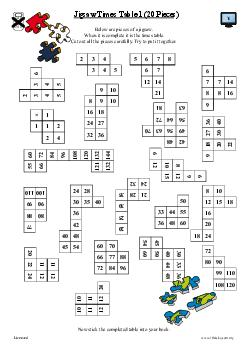Jigsaw Times Table 1 (20 Pieces)
Number and Algebra
Comment: 4.2.3
2 / 40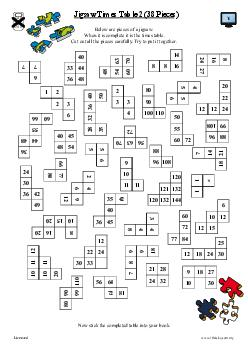Jigsaw Times Table 2 (38 peices)
Number and Algebra
Comment: 4.2.4
3 / 40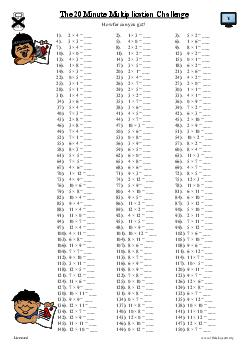The 20 Minute Multiplication Challenge
Number and Algebra
Comment: 4.2.5
4 / 40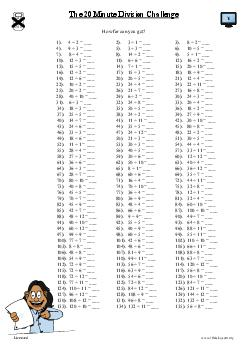The 20 Minute Division challenge
Number and Algebra
Comment: 4.2.6
5 / 40Multiplication and Division Problems (a)
Number and Algebra
Comment: 4.2.7
6 / 40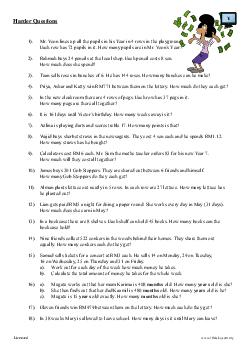Multiplication and Division Problems (b)
Number and Algebra
Comment: 4.2.8
7 / 40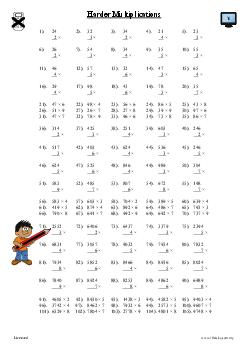Harder Multiplications
Number and Algebra
Comment: 4.2.9
8 / 40Harder Divisions
Number and Algebra
Comment: 4.2.10
9 / 40The 20 Minute Multiplication and Division Challenge
Number and Algebra
Comment: 4.2.11
10 / 40More Division Revision
Number and Algebra
Comment: 4.2.12
11 / 40Word Search 1. (Multiplication and Division)
Number and Algebra
Comment: 4.2.13
12 / 40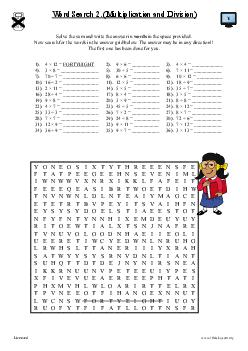Word Search 2. (Multiplication and Division)
Number and Algebra
Comment: 4.2.14
13 / 40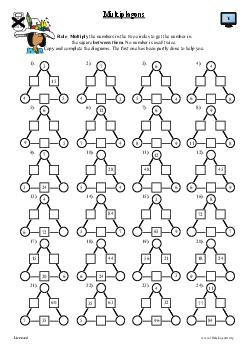Multiplagons (a)
Number and Algebra
Comment: 4.2.15
14 / 40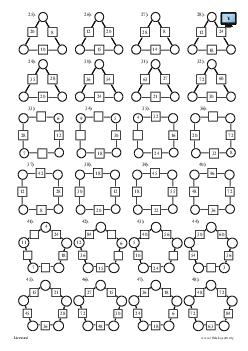Multiplagons (b)
Number and Algebra
Comment: 4.2.16
15 / 40

Multiplication Grids (a)
Number and Algebra
Comment: 4.2.17
16 / 40

Multiplication Grids (b)
Number and Algebra
Comment: 4.2.18
17 / 40

Four in a Line-Multiplication Mania
Number and Algebra
Comment: 4.2.21
18 / 40

Multiple Maze (2, 3, 4 & 5)
Number and Algebra
Comment: 4.2.26
19 / 40

Multiple Maze (6, 7, 8 & 9)
Number and Algebra
Comment: 4.2.30
20 / 40

Some Products (a)
Number and Algebra
Comment: 4.2.33
21 / 40

Some Products (b)
Number and Algebra
Comment: 4.2.34
22 / 40

Number Hexagon Puzzle Grid
Number and Algebra
Comment: 4.2.35
23 / 40

Diabolical Magic Squares (a)
Number and Algebra
Comment: 4.2.39
24 / 40

Diabolical Magic Squares (b)
Number and Algebra
Comment: 4.2.40
25 / 40

The Odd Pyramid (a)
Number and Algebra
Comment: 4.2.41
26 / 40

The Odd Pyramid (b)
Number and Algebra
Comment: 4.2.42
27 / 40

Factors
Number and Algebra
Comment: 4.2.22
28 / 40

Number Golf (Six Hole Course)
Number and Algebra
Comment: 4.2.25
29 / 40

Multiplication Puzzles
Number and Algebra
Comment: 4.2.31
30 / 40

Triangle
Number and Algebra
Comment: 4.2.20
31 / 40Multiple Maze (3, 4, 5 & 6)
Number and Algebra
Comment: 4.2.27
32 / 40Multiple Maze (4, 5, 6 & 7)
Number and Algebra
Comment: 4.2.28
33 / 40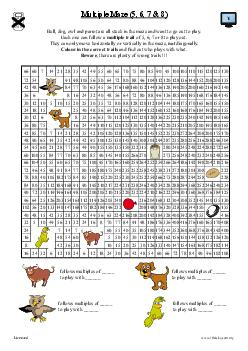Multiple Maze (5, 6, 7 & 8)
Number and Algebra
Comment: 4.2.29
34 / 40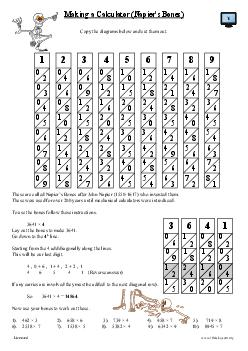Making a Calculator (Napier's Bones)
Number and Algebra
Comment: 4.2.32
35 / 40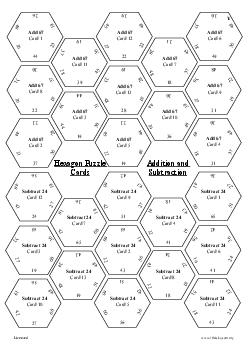Hexagon Puzzle Cards (Addition and Subtraction)
Number and Algebra
Comment: 4.2.36
36 / 40Hexagon Puzzle Cards (Multiplication and Divisions)
Number and Algebra
Comment: 4.2.37
37 / 40Hex-a Multiplying Game
Number and Algebra
Comment: 4.2.19
38 / 40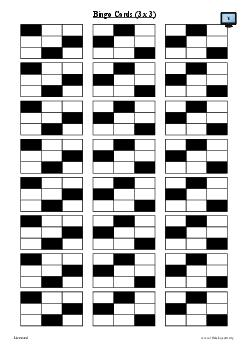Bingo Cards (3 x 3)
Number and Algebra
Comment: 4.2.23
39 / 40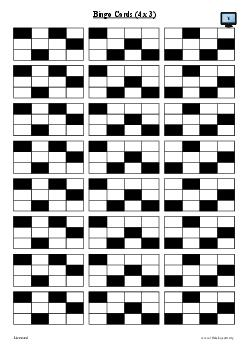Bingo Cards (4 x 3)
Number and Algebra
Comment: 4.2.24
40 / 40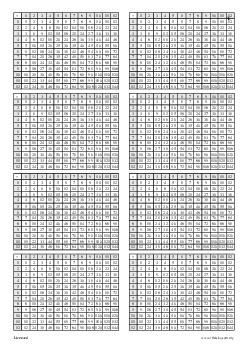Multiplication Grids
Number and Algebra
Comment: 4.2.38